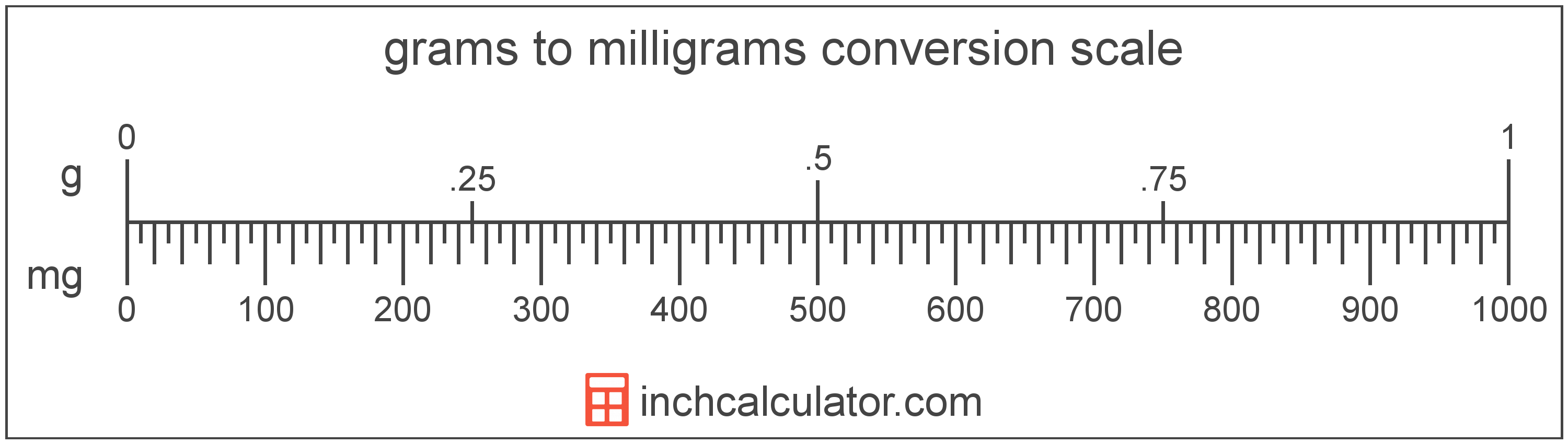# Milligrams to Grams Conversion

Enter the weight in milligrams below to get the value converted to grams.

Results in Grams:1 mg = 0.001 g
Do you want to convert grams to milligrams?

## How to Convert Milligrams to GramsTo convert a milligram measurement to a gram measurement, divide the weight by the conversion ratio.

Since one gram is equal to 1,000 milligrams, you can use this simple formula to convert:

grams = milligrams ÷ 1,000

The weight in grams is equal to the milligrams divided by 1,000.

For example, here's how to convert 5,000 milligrams to grams using the formula above.
5,000 mg = (5,000 ÷ 1,000) = 5 g

## Milligrams

The milligram is the mass equal to 1/1,000 of a gram, or 0.0154 grains.

The milligram, or milligramme, is an SI unit of weight in the metric system. In the metric system, "milli" is the prefix for 10-3. Milligrams can be abbreviated as mg; for example, 1 milligram can be written as 1 mg.

## Grams

A gram is the mass/weight equal to 1/1,000 of a kilogram and is roughly equivalent to the mass of one cubic centimeter of water.

The gram, or gramme, is an SI unit of weight in the metric system. Grams can be abbreviated as g; for example, 1 gram can be written as 1 g.

## Milligram to Gram Conversion Table

Milligram measurements converted to grams
Milligrams Grams
1 mg 0.001 g
2 mg 0.002 g
3 mg 0.003 g
4 mg 0.004 g
5 mg 0.005 g
6 mg 0.006 g
7 mg 0.007 g
8 mg 0.008 g
9 mg 0.009 g
10 mg 0.01 g
20 mg 0.02 g
30 mg 0.03 g
40 mg 0.04 g
50 mg 0.05 g
60 mg 0.06 g
70 mg 0.07 g
80 mg 0.08 g
90 mg 0.09 g
100 mg 0.1 g
200 mg 0.2 g
300 mg 0.3 g
400 mg 0.4 g
500 mg 0.5 g
600 mg 0.6 g
700 mg 0.7 g
800 mg 0.8 g
900 mg 0.9 g
1,000 mg 1 g